Electric Forces and Fields Holt Chapter 16 Reading

• Slides: 60Electric Forces and Fields Holt Chapter 16 Reading this chapter is strongly recommended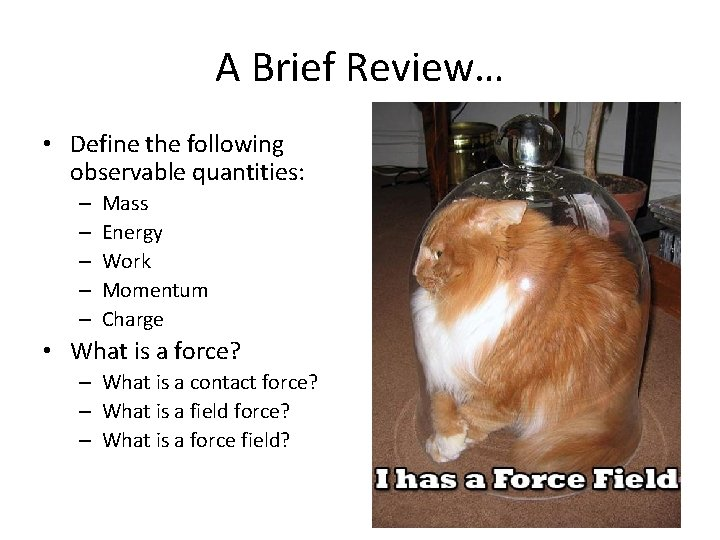A Brief Review… • Define the following observable quantities: – – – Mass Energy Work Momentum Charge • What is a force? – What is a contact force? – What is a field force? – What is a force field?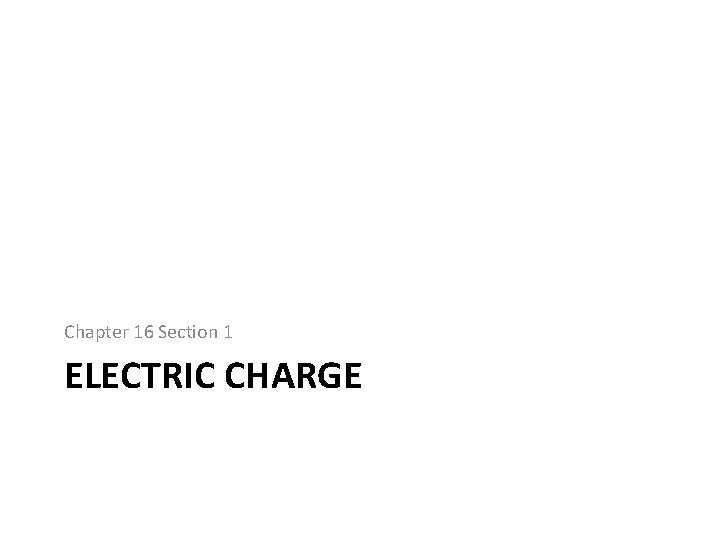Chapter 16 Section 1 ELECTRIC CHARGE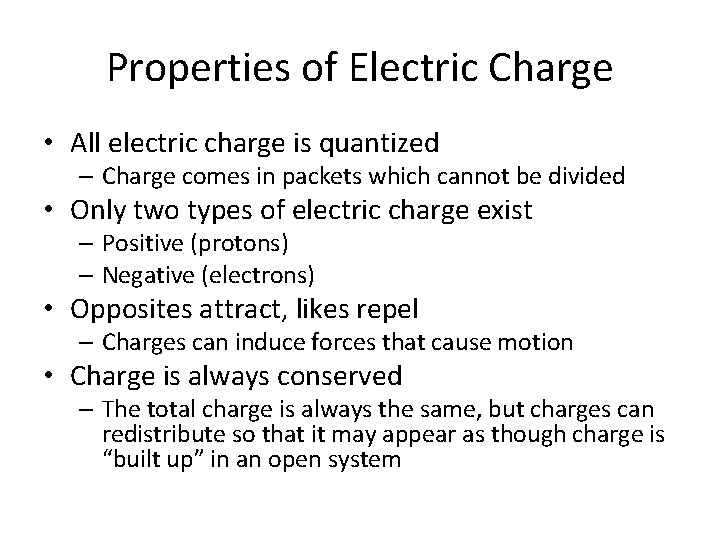Properties of Electric Charge • All electric charge is quantized – Charge comes in packets which cannot be divided • Only two types of electric charge exist – Positive (protons) – Negative (electrons) • Opposites attract, likes repel – Charges can induce forces that cause motion • Charge is always conserved – The total charge is always the same, but charges can redistribute so that it may appear as though charge is “built up” in an open systemAll Electric Charge is Quantized • You can only exchange charge in discrete packets, although it is usually transferred in batches of these packets – The smallest unit of charge is the amount of charge carried by a single electron (or proton) – Quantized charge is analogous to our use of money: we can transfer large sums of money, but those large amounts are just lots of pennies • All charge is a multiple of a fundamental unit of charge, symbolized by e – Electrons �–e – Protons �+e – The SI unit of charge is the Coulomb (C) • e = 1. 6 x 10 -19 CAll Electric Charge is Quantized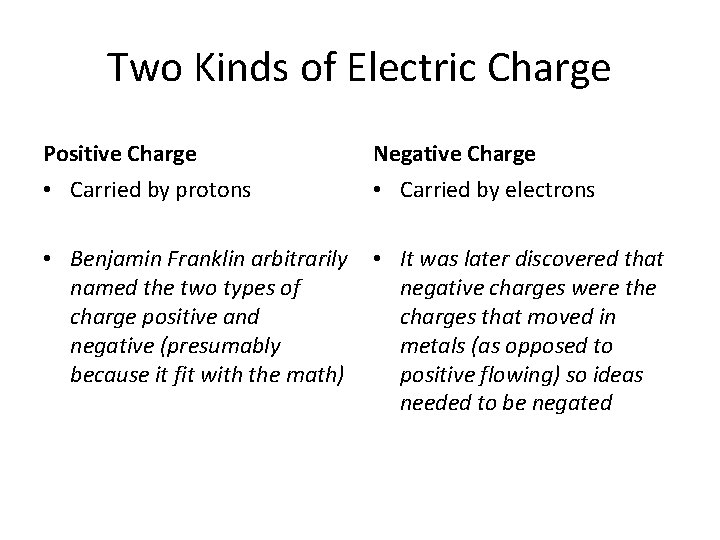Two Kinds of Electric Charge Positive Charge Negative Charge • Carried by protons • Carried by electrons • Benjamin Franklin arbitrarily named the two types of charge positive and negative (presumably because it fit with the math) • It was later discovered that negative charges were the charges that moved in metals (as opposed to positive flowing) so ideas needed to be negated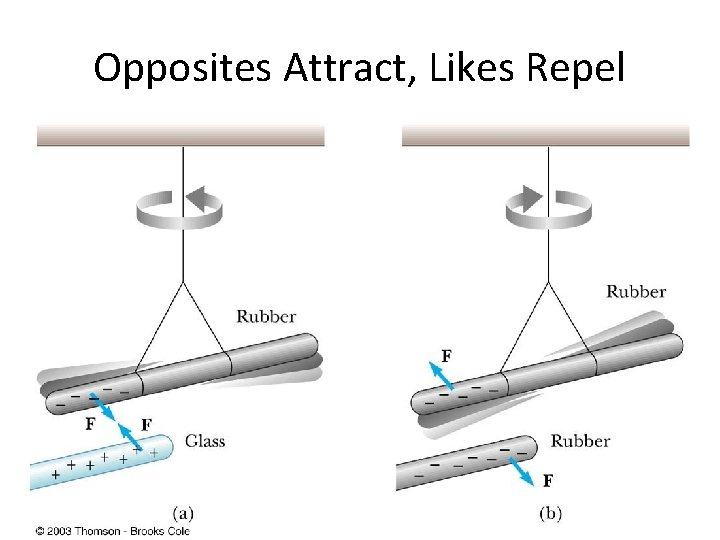Opposites Attract, Likes RepelCharges are Always Conserved • Charge is carried by mass (electrons or protons) and since mass must be conserved, charge must be conserved • Charge can be “built up” in an area by collecting a lot of particles of similar charge in an area – Think of building a sand castle in a sandbox: you move the sand around to make a pile, without changing the total amount of sand in the boxTransfer of Electric Charge • There are occasions where electric charge of one type or another will build up in an area, and this can only be achieved by the movement of charged particles – Particles of similar type must move to the area to build the charge – Particles must be able to leave the area to dissipate the charge • Ancient Greeks observed electric and magnetic phenomena as early as 700 BC • Found that amber, when rubbed, became “electrified” and attracted pieces of straw or feathersConductors • Conductors are materials in which the electric charges move freely – Copper, aluminum and silver are good conductors – When a conductor is charged in a small region, the charge readily distributes itself over the entire surface of the materialInsulators • Insulators are materials in which electric charges do not move freely – Glass and rubber are examples of insulators – When insulators are charged by rubbing, only the rubbed area becomes charged • There is no tendency for the charge to move into other regions of the material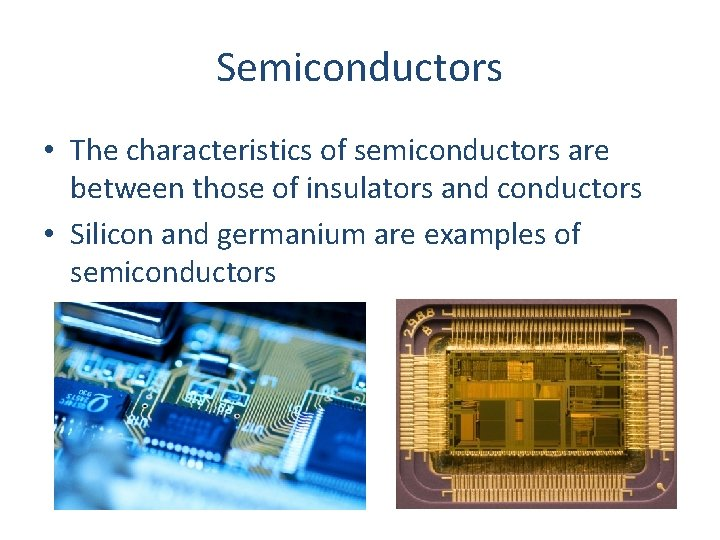Semiconductors • The characteristics of semiconductors are between those of insulators and conductors • Silicon and germanium are examples of semiconductorsHair Raising Electrostatic! • Why are all of the hairs standing on end, in defiance of gravity? – There is a large buildup of the same charge on the hairs, so the hairs repel and the electric force is enough to overcome gravitation. • Can you tell by this if the person and their hair are conductors or insulators? If so, which are they? – Why could this be?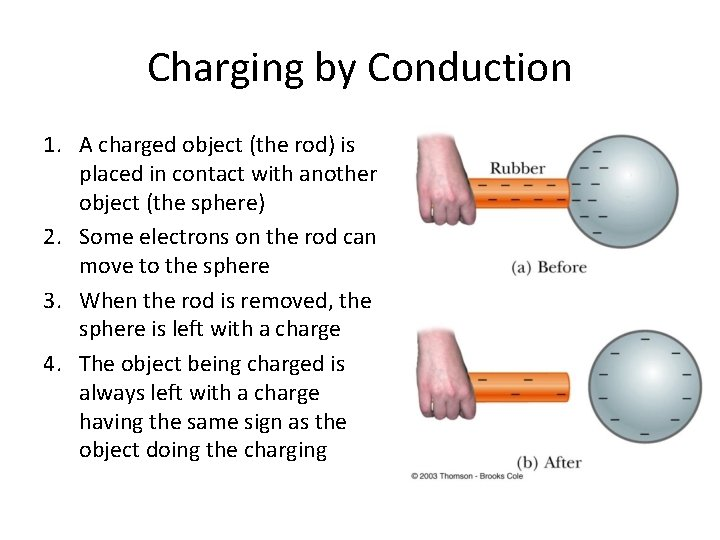Charging by Conduction 1. A charged object (the rod) is placed in contact with another object (the sphere) 2. Some electrons on the rod can move to the sphere 3. When the rod is removed, the sphere is left with a charge 4. The object being charged is always left with a charge having the same sign as the object doing the chargingCharging by Induction 1. A negatively charged rubber rod is brought near an uncharged sphere 2. The charges in the sphere are redistributed – Some of the electrons in the sphere are repelled from the electrons in the rod 3. The wire to ground is removed, the sphere is left with an excess of induced positive charge 4. The positive charge on the sphere is evenly distributed due to the repulsion between the positive charges • Charging by induction requires no contact with the object inducing the chargePolarization • In the presence of a charged object, these centers may separate slightly – This results in more positive charge on one side of the molecule than on the other side • This realignment of charge on the surface of an insulator is known as polarization – The paper is polarized by the charged plastic comb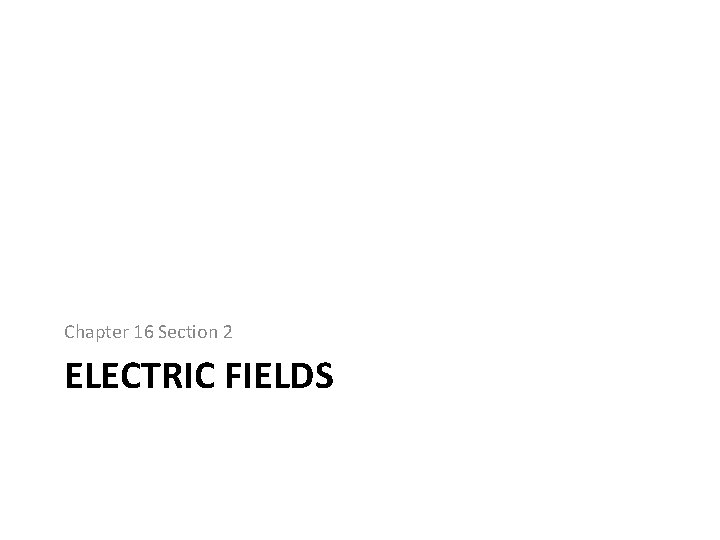Chapter 16 Section 2 ELECTRIC FIELDS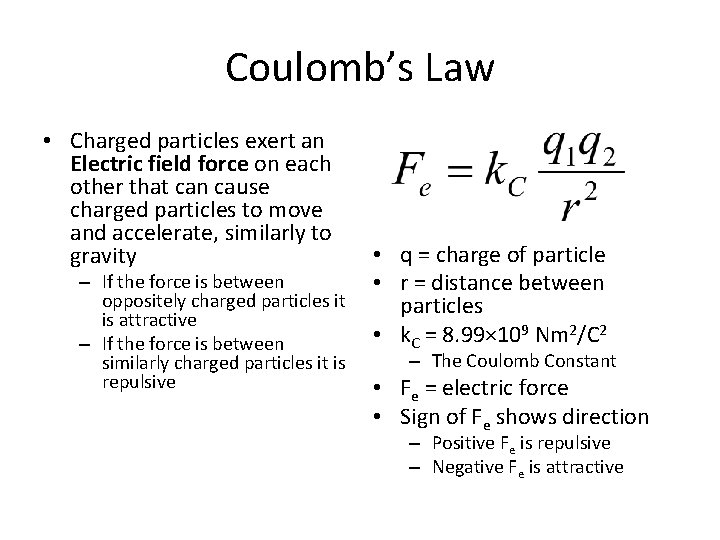Coulomb’s Law • Charged particles exert an Electric field force on each other that can cause charged particles to move and accelerate, similarly to gravity – If the force is between oppositely charged particles it is attractive – If the force is between similarly charged particles it is repulsive • q = charge of particle • r = distance between particles • k. C = 8. 99× 109 Nm 2/C 2 – The Coulomb Constant • Fe = electric force • Sign of Fe shows direction – Positive Fe is repulsive – Negative Fe is attractive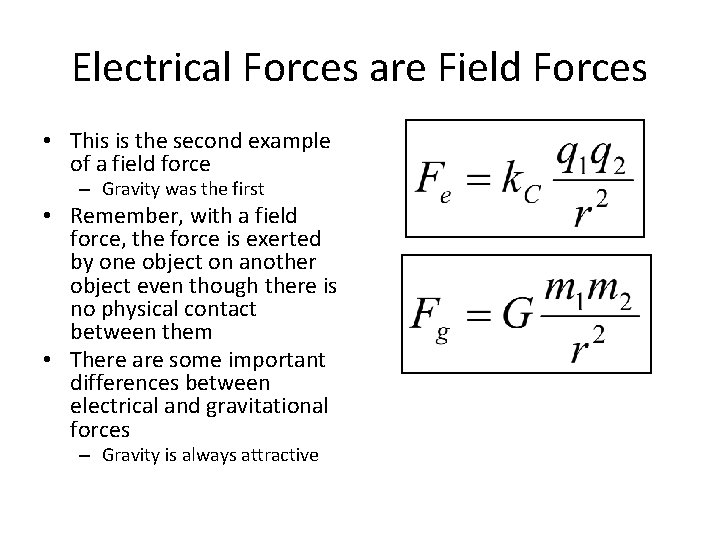Electrical Forces are Field Forces • This is the second example of a field force – Gravity was the first • Remember, with a field force, the force is exerted by one object on another object even though there is no physical contact between them • There are some important differences between electrical and gravitational forces – Gravity is always attractiveTwo electrostatic point charges of +3 C and +4 C exert a force on each other of 175 N. Is the force attractive or repulsive? What is the distance between the two charges? Attractive or Repulsive?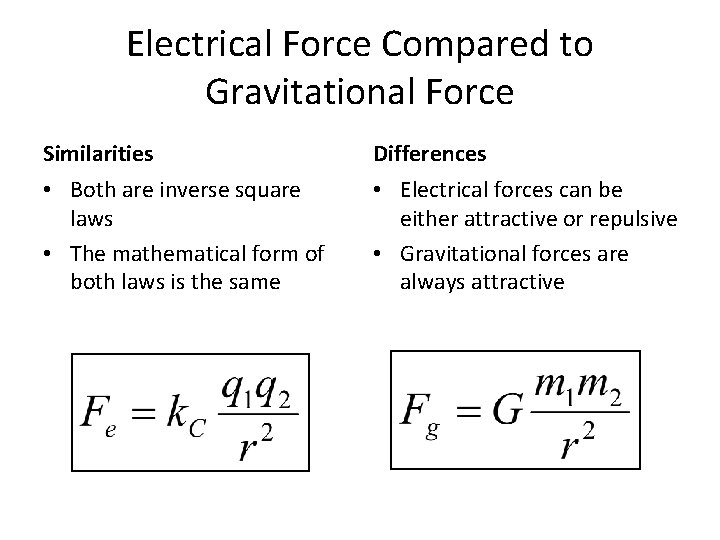Electrical Force Compared to Gravitational Force Similarities Differences • Both are inverse square laws • The mathematical form of both laws is the same • Electrical forces can be either attractive or repulsive • Gravitational forces are always attractive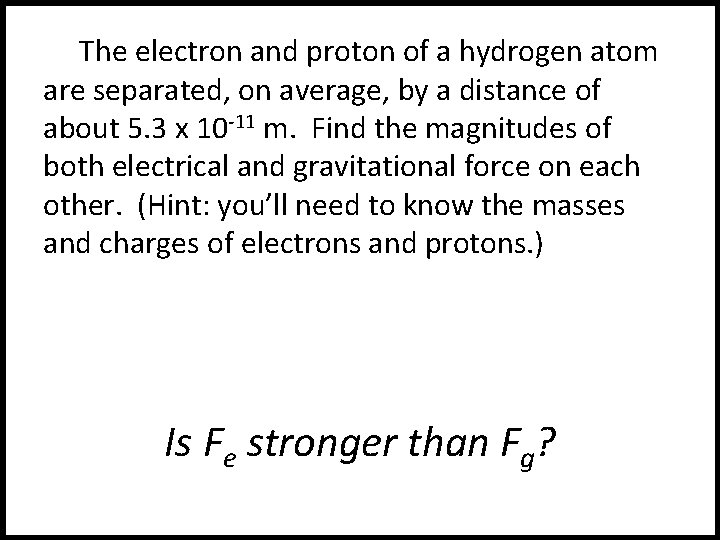The electron and proton of a hydrogen atom are separated, on average, by a distance of about 5. 3 x 10 -11 m. Find the magnitudes of both electrical and gravitational force on each other. (Hint: you’ll need to know the masses and charges of electrons and protons. ) Is Fe stronger than Fg?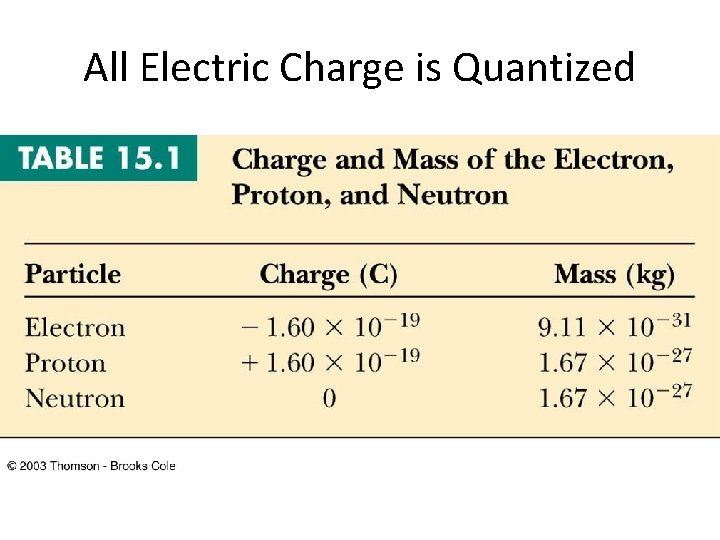All Electric Charge is Quantized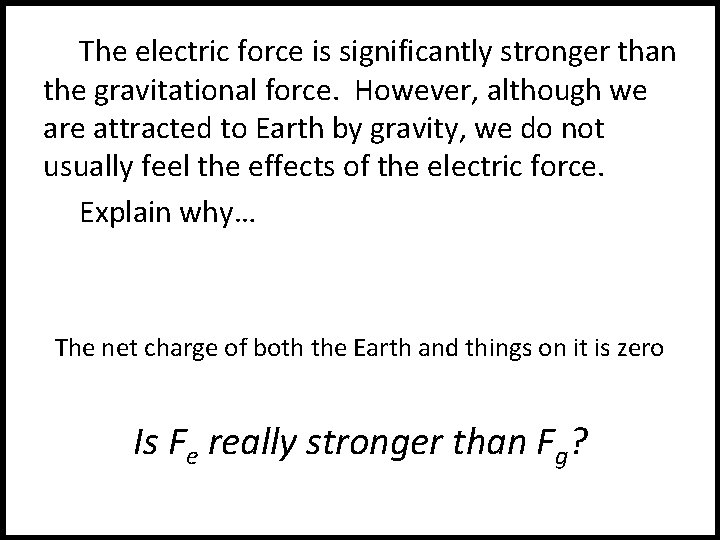The electric force is significantly stronger than the gravitational force. However, although we are attracted to Earth by gravity, we do not usually feel the effects of the electric force. Explain why… The net charge of both the Earth and things on it is zero Is Fe really stronger than Fg?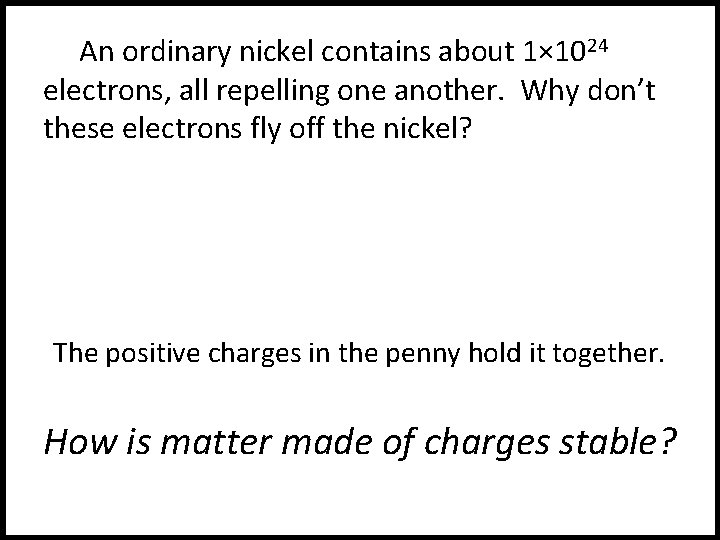An ordinary nickel contains about 1× 1024 electrons, all repelling one another. Why don’t these electrons fly off the nickel? The positive charges in the penny hold it together. How is matter made of charges stable?When the distance between two negatively charged balloons is doubled, by what factor does the repulsive force between them change? It is weakened to ¼ its original strength. How does distance effect the Fe?Vector Nature of Electric Forces • Two point charges are separated by a distance r • The like charges produce a repulsive force between them • The force on q 1 is equal in magnitude and opposite in direction to the force on q 2Vector Nature of Forces • Two point charges are separated by a distance r • The unlike charges produce a attractive force between them • The force on q 1 is equal in magnitude and opposite in direction to the force on q 2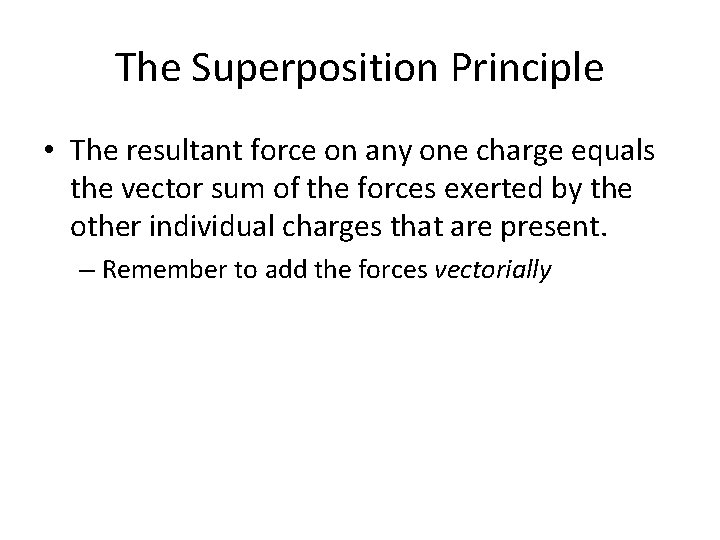The Superposition Principle • The resultant force on any one charge equals the vector sum of the forces exerted by the other individual charges that are present. – Remember to add the forces vectorially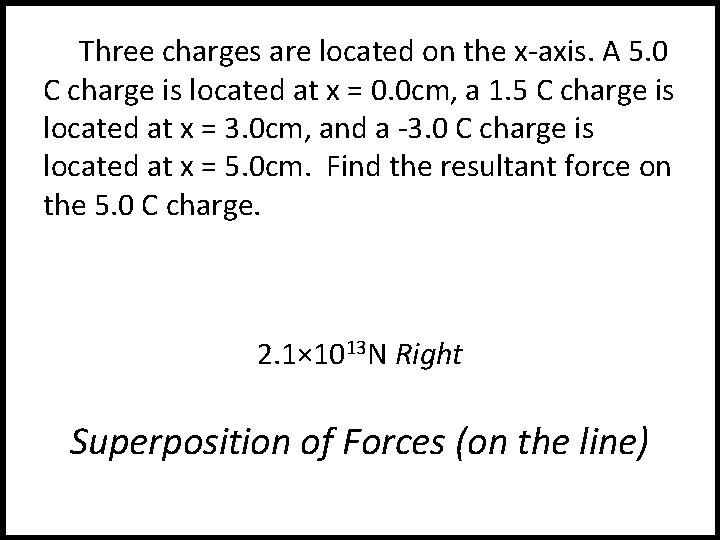Three charges are located on the x-axis. A 5. 0 C charge is located at x = 0. 0 cm, a 1. 5 C charge is located at x = 3. 0 cm, and a -3. 0 C charge is located at x = 5. 0 cm. Find the resultant force on the 5. 0 C charge. 2. 1× 1013 N Right Superposition of Forces (on the line)Three charges lie along the x-axis. One positive charge, q 1 = 15μC, is at the origin, and another positive charge, q 2 = 6μC, is at the at x=1. 0 m. The third charge is at x=2. 5 m and has a charge of -3μC. What is the net force felt by the second charge (q 2) at the described location? Superposition of Forces (on the line)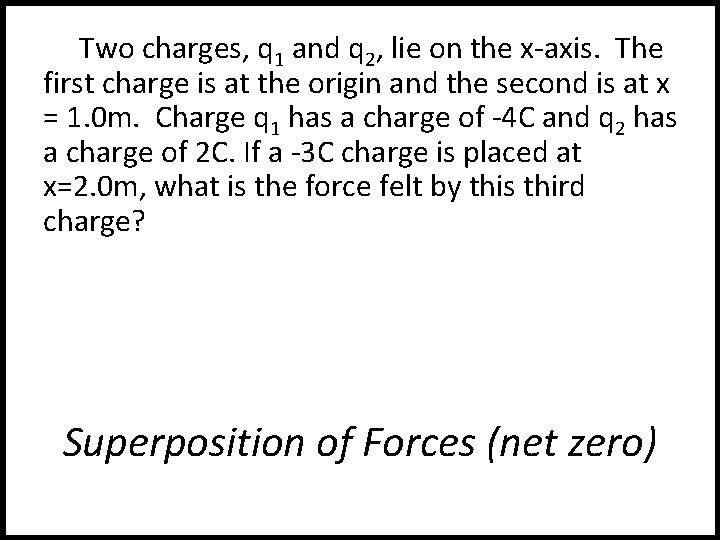Two charges, q 1 and q 2, lie on the x-axis. The first charge is at the origin and the second is at x = 1. 0 m. Charge q 1 has a charge of -4 C and q 2 has a charge of 2 C. If a -3 C charge is placed at x=2. 0 m, what is the force felt by this third charge? Superposition of Forces (net zero)Chapter 16 Section 3 THE ELECTRIC FIELDElectric Field Strength • James Clerk Maxwell developed an approach to discussing fields • An electric field is said to exist in the region of space around a charged object – When another charged object enters this electric field, the field exerts a force on the second charged object Gravity FieldElectric Field Strength • A charged particle, with charge Q, produces an electric field in the region of space around it • A small test charge, qo, placed in the field, will experience a force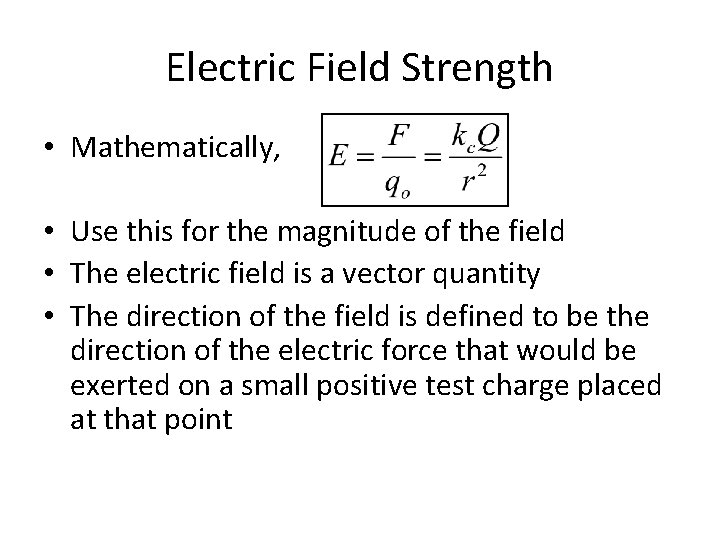Electric Field Strength • Mathematically, • Use this for the magnitude of the field • The electric field is a vector quantity • The direction of the field is defined to be the direction of the electric force that would be exerted on a small positive test charge placed at that point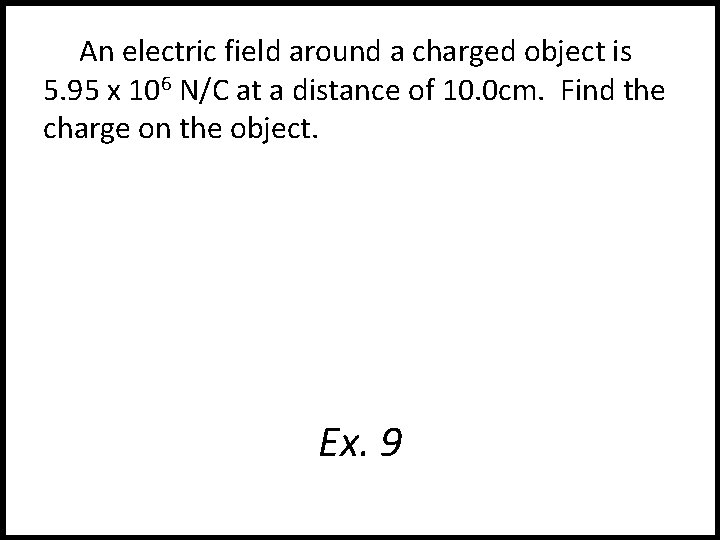An electric field around a charged object is 5. 95 x 106 N/C at a distance of 10. 0 cm. Find the charge on the object. Ex. 9Direction of Electric Field • The electric field produced by a negative charge is directed toward the charge – A positive test charge would be attracted to the negative source chargeDirection of Electric Field, cont • The electric field produced by a positive charge is directed away from the charge – A positive test charge would be repelled from the positive source charge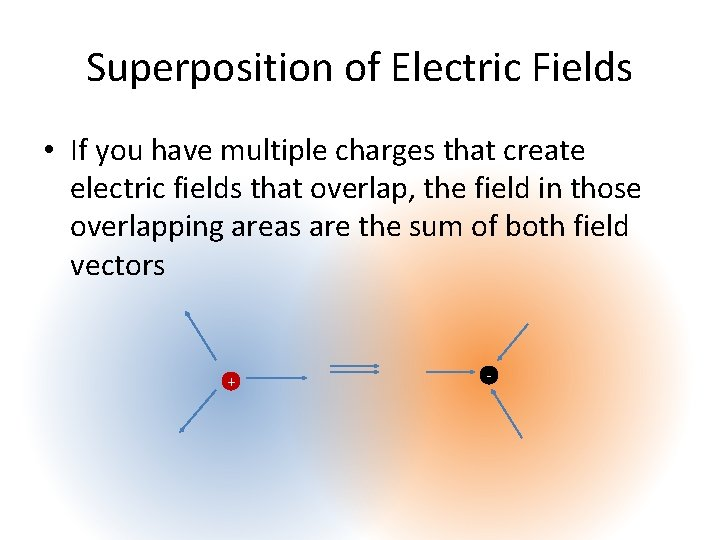Superposition of Electric Fields • If you have multiple charges that create electric fields that overlap, the field in those overlapping areas are the sum of both field vectors + -A charge q 1 = 7 C is at the origin, and a charge q 2 = -5 C is on the x-axis. 3 m from the origin. Find the electric field strength at point P, which is on the y-axis 0. 400 m from the origin. Ex. 9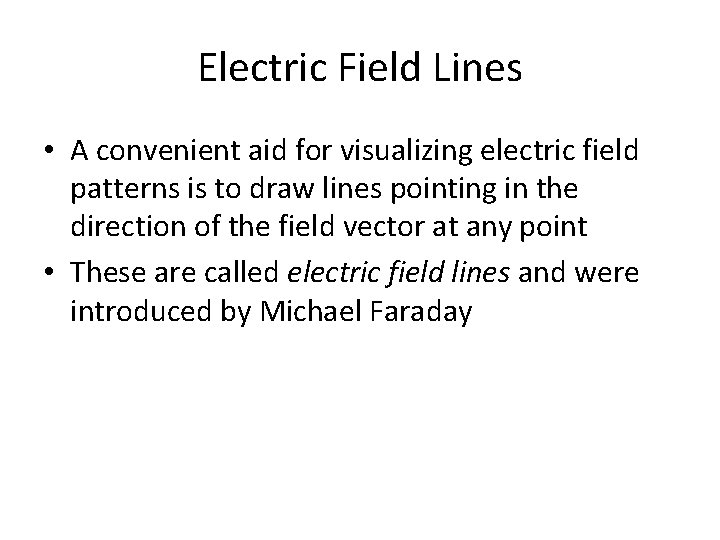Electric Field Lines • A convenient aid for visualizing electric field patterns is to draw lines pointing in the direction of the field vector at any point • These are called electric field lines and were introduced by Michael Faraday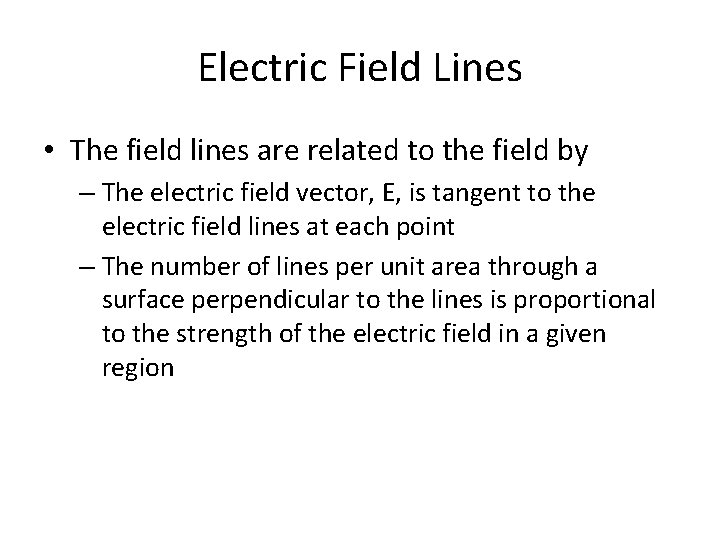Electric Field Lines • The field lines are related to the field by – The electric field vector, E, is tangent to the electric field lines at each point – The number of lines per unit area through a surface perpendicular to the lines is proportional to the strength of the electric field in a given regionElectric Field Line Patterns • Point charge • The lines radiate equally in all directions • For a positive source charge, the lines will radiate outward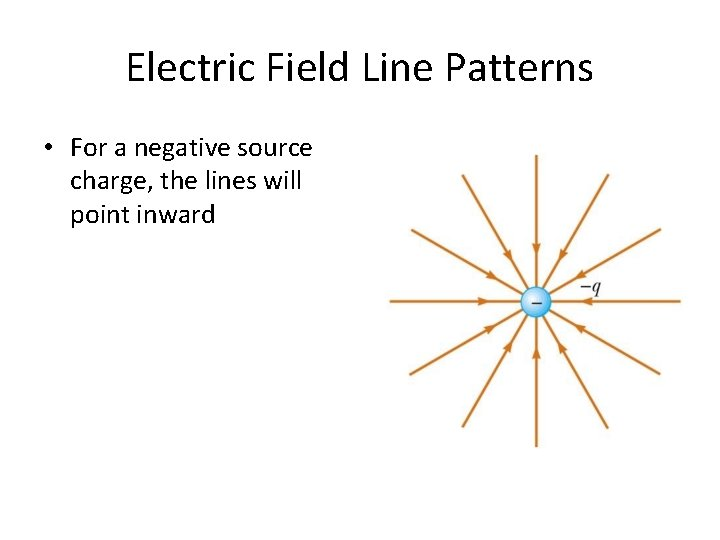Electric Field Line Patterns • For a negative source charge, the lines will point inwardElectric Field Line Patterns • An electric dipole consists of two equal and opposite charges • The high density of lines between the charges indicates the strong electric field in this region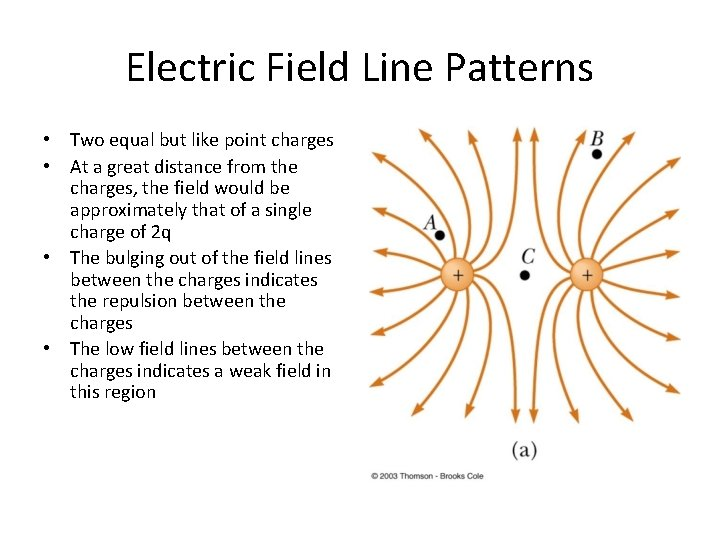Electric Field Line Patterns • Two equal but like point charges • At a great distance from the charges, the field would be approximately that of a single charge of 2 q • The bulging out of the field lines between the charges indicates the repulsion between the charges • The low field lines between the charges indicates a weak field in this regionRules for Drawing Electric Field Lines • The lines for a group of charges must begin on positive charges and end on negative charges – In the case of an excess of charge, some lines will begin or end infinitely far away • The number of lines drawn leaving a positive charge or ending on a negative charge is proportional to the magnitude of the charge • No two field lines can cross each otherQUICK QUIZ 15. 7 Rank the magnitudes of the electric field at points A, B, and C in the figure below, largest magnitude first.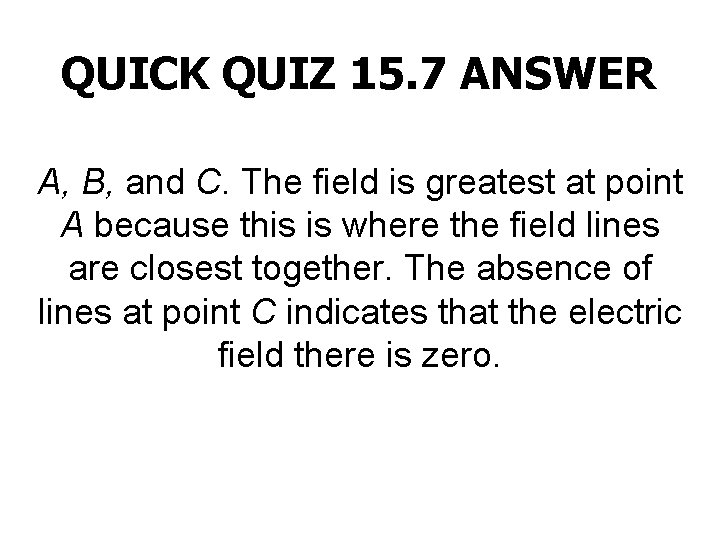QUICK QUIZ 15. 7 ANSWER A, B, and C. The field is greatest at point A because this is where the field lines are closest together. The absence of lines at point C indicates that the electric field there is zero.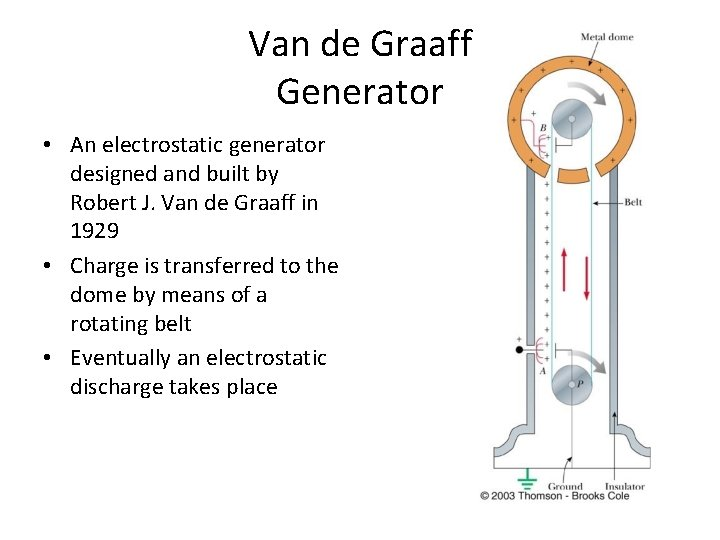Van de Graaff Generator • An electrostatic generator designed and built by Robert J. Van de Graaff in 1929 • Charge is transferred to the dome by means of a rotating belt • Eventually an electrostatic discharge takes place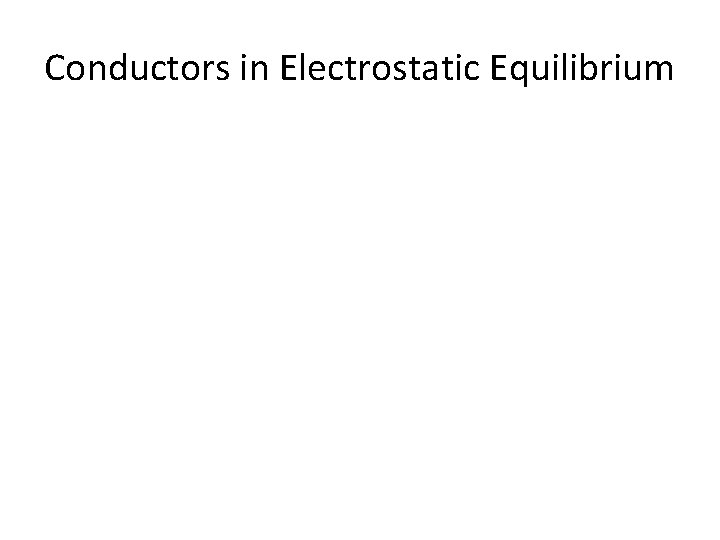Conductors in Electrostatic EquilibriumHolt Chapter 17 Section 1 ELECTRIC POTENTIAL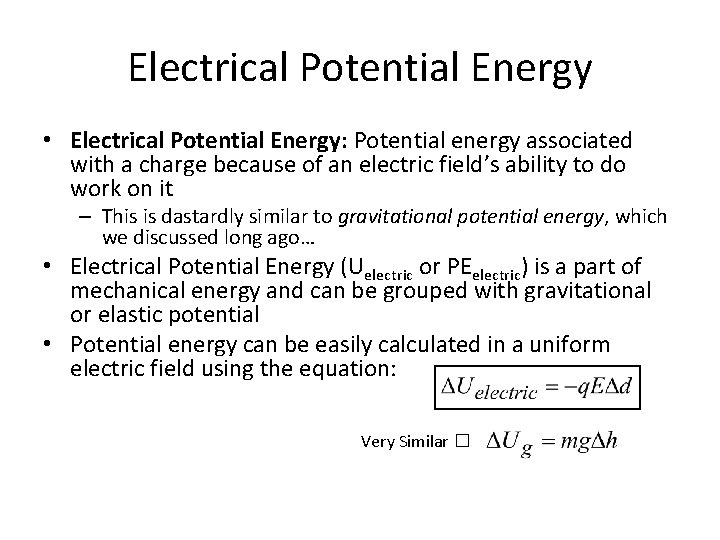Electrical Potential Energy • Electrical Potential Energy: Potential energy associated with a charge because of an electric field’s ability to do work on it – This is dastardly similar to gravitational potential energy, which we discussed long ago… • Electrical Potential Energy (Uelectric or PEelectric) is a part of mechanical energy and can be grouped with gravitational or elastic potential • Potential energy can be easily calculated in a uniform electric field using the equation: Very Similar �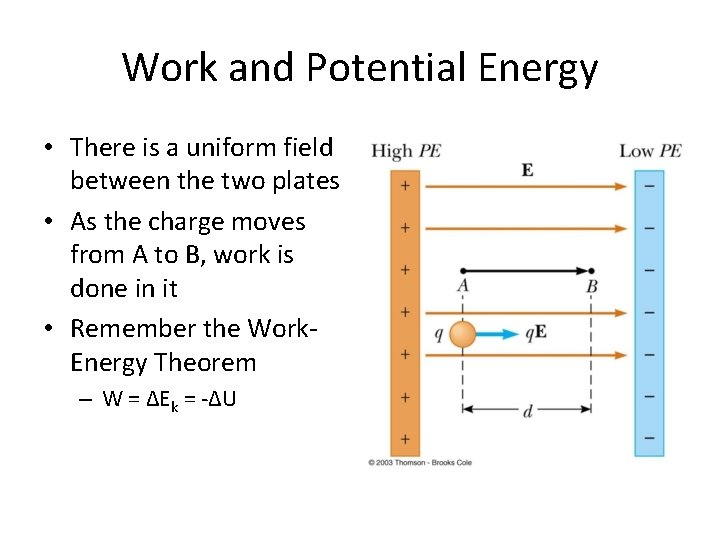Work and Potential Energy • There is a uniform field between the two plates • As the charge moves from A to B, work is done in it • Remember the Work. Energy Theorem – W = ΔEk = -ΔU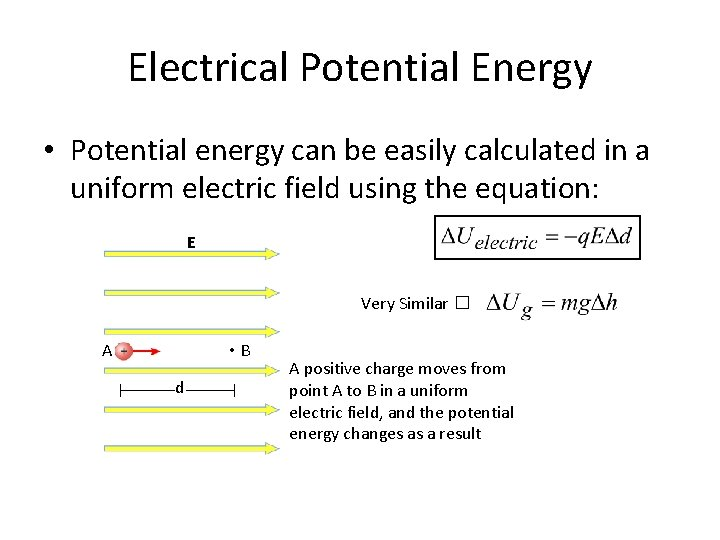Electrical Potential Energy • Potential energy can be easily calculated in a uniform electric field using the equation: E Very Similar � A • B d A positive charge moves from point A to B in a uniform electric field, and the potential energy changes as a resultBlue Border Slides are ‘brain candy’ and not necessary notes A Quick Proof of Electrical Potential Energy and Analogy to Ug • The Equation for Electrical Potential Energy has some surprising roots: 1. 2. 3. 4. 5. 6. The Work-Energy Theorem� The Definition of Work� Redefine Work with F=ma� Sub -ΔU for work� The basic units are analogous � Gravity & E-fields are analogous �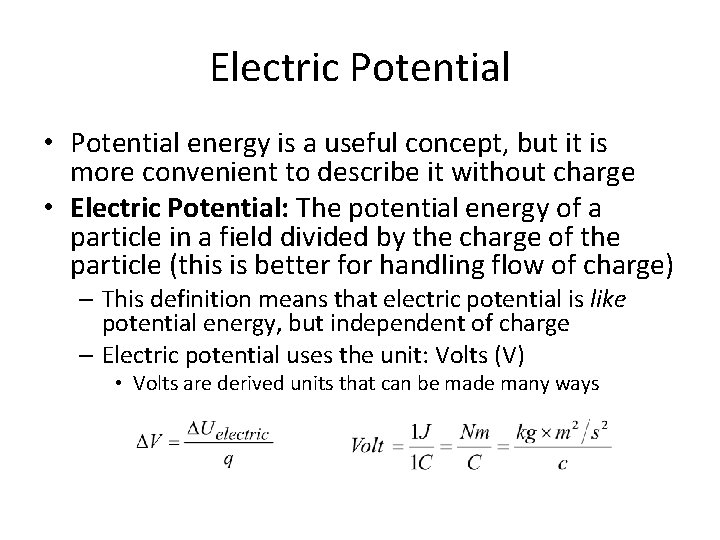Electric Potential • Potential energy is a useful concept, but it is more convenient to describe it without charge • Electric Potential: The potential energy of a particle in a field divided by the charge of the particle (this is better for handling flow of charge) – This definition means that electric potential is like potential energy, but independent of charge – Electric potential uses the unit: Volts (V) • Volts are derived units that can be made many ways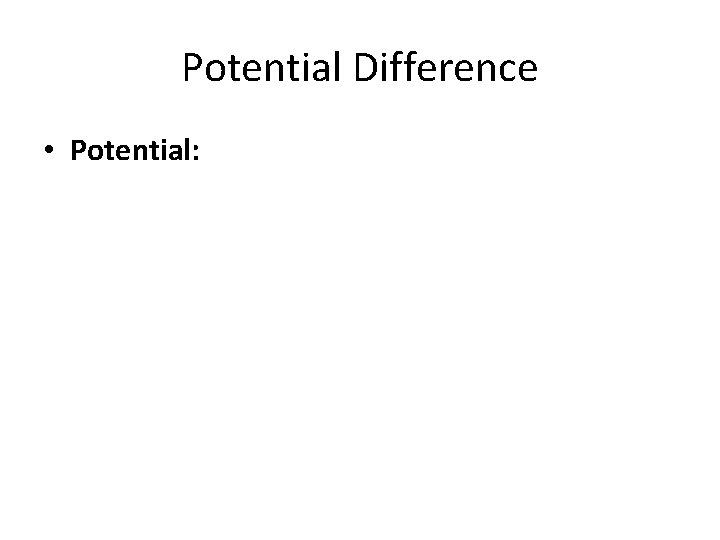Potential Difference • Potential: